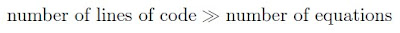## Field of Science

• 1 week ago in The Phytophactor
• 4 weeks ago in Angry by Choice
• 9 months ago in Chinleana
• 1 year ago in Field Notes
• 2 years ago in Moss Plants and More
• 3 years ago in Rule of 6ix
• 3 years ago in Games with Words
• 3 years ago in Epiphenom
• 4 years ago in Protein Evolution and Other Musings
• 4 years ago in The View from a Microbiologist
• 5 years ago in Memoirs of a Defective Brain
• 7 years ago in Sex, Genes & Evolution
• 8 years ago in Life of a Lab Rat
• 8 years ago in Disease Prone
• in The Biology Files

### Semi-analytical or semi-numericalSome people think that asking whether the glass is half full or half empty is a deep and telling question; these people may also think that banal is a country. Scientists also play this little game sometimes when describing their work. Some science is numerical (of or pertaining to numbers; of the nature of a number). Some science is analytical (pertaining to or proceeding by analysis). Many scientists claim that their work is semi-analytical or semi-numerical. The prefix semi may be defined as precisely half (as per its original etymology), but here it must be used meaning partially or quasi. These definitions are useless because in the context of the 'hard' sciences, every shred of research is based on numbers (so it must be numerical) and every number is scrutinized and extrapolated (so it must be analytical) to relevant cases with theory. In conclusion all work is numerical and analytical, but it can't be 100% either so all work is semi-analytical and semi-numerical. Thus the actual labeling of research under one category or the other is useless.

In colloquial scientific usage numerical seems to apply when the research has reached some arbitrary threshold where the number of lines of code is much greater than the number of equations used. So maybe numerical meansExcept, this definition seems necessary but not sufficient because if your doing observations then you probably also meet this criteria doing the data reduction. So really, numerical means that the result you derive cannot be proven with a single succinct analytical equation (though the correlations found may be analytical the proof of such correlations cannot be proven analytically). For example the four color theorem has only been proven using a computer-assisted proof. Some mathematicians fundamentally object to putting their trust in computers over the logical deductions of humans, and yet the four color theorem stands proven only by computers.And so colloquially most scientists would be content to say that the four-color theorem or simulations (thought the comparison of these kinds of research together is dubious) done in the physical sciences are numerical. Scientists seem to think their research leans one direction or another on the analytical/numerical spectrum (also, I have no idea if this is standard or just my interpretation, but as I have seen it research is normally described as being semi with respect to the lesser used approach; that is research that is primarily analytic and only uses a tiny bit of numerical work is described as semi-numerical), but the distinction seems delicate given accepted modern scientific approaches . Upon cursory examination it is all semi-numerical or semi-analytical and the difference well the difference makes no difference all, but that isn't true is it?

1.My proof (*) of the four color theorem enable one to state that the four color theorem has been proven without of the use of a computer assistance. Unfortunately there are some mathematicians even without a proof saying that four color theorem can only be proven using a computer. I think this is because of the strange history of the four color problem. Therefore I place it just in the middle e.g., the glass is half full and half empty.

Cahit

(*) I. Cahit, A Victorian Age Proof of the Four Color Theorem, http://arxiv.org/abs/0903.4108

2.Another interesting aside is whether or not analytic descriptions can yield the same level of understanding of nature when compared to numerical descriptions. The ancient Chinese considered Astronomy a full time career. They recorded the positions of the planets to high accuracy, but never came up with Kepler's simple analytic laws.

3.Cahit your proof of the four color theorem is intriguing. I don't know if I understand it fully. I need to re-post about the four color theorem. For interested readers, there are some online explanations of the theorem and proofs here, and here. I am wondering if there are there any publications with the spiral proof you demonstrate?

4.Spiral chain coloring (direct algorithmic) proof of the 4CT has been first announced at ICM2006,Madrid.
See http://www.icm2006.org/v_f/AbsDef/Shorts/abs_0003.pdf"

I hope it will also appear as a book chapter in 2010.

http://www.springer.com/birkhauser/mathematics/book/978-0-8176-4788-9?detailsPage=toc

Summary of the proofs has been presented also in a conference Ankara Mathematics Days held in Middle East Technical University, 4-5 June 2009.